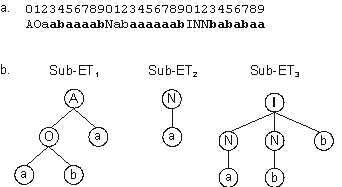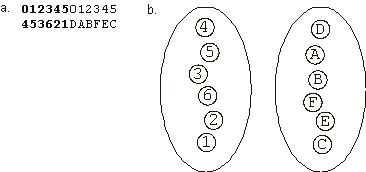Home
News
Author
Q&A
Tutorials
GEP Biblio
Contacts

Visit Gepsoft

Gene Expression Programming: Mathematical Modeling by an Artificial Intelligence

Information decoding: Translation

From the simplest individual to the most complex, the expression of genetic information in GEP starts with translation. An overview of translation has already been presented in section 2.1.1, where we learned how to decode K-expressions. Since then, we have learned about minor variants of chromosomal organization, each requiring different levels and different paths of expression. However, in all cases, the first step in the expression of the genetic information consists in the construction of sub-ETs.

Consider the following single-gene chromosome (the tail is shown in blue):

 0123456789012345 NIAbObbaaaabaabb (2.17)

The symbols {A, O, N, I} represent, respectively, the Boolean functions AND, OR, NOT, and IF, where the first two take two arguments, NOT takes one argument, and the last one is a function of three arguments. In this case, the product of translation is the following ET with nine elements:For this simple individual, a single unit ET is the “organism” and, in this case, the expression of the genetic information ends with translation. Note that this particular program is a solution to the NOR function.

When the genome contains more than one gene, each gene is independently translated as a sub-ET. For instance, the following three-genic chromosome codes for the three sub-ETs shown in Figure 2.6 (the tails are shown in blue):

 012345678901234567890123456789 AOaabaaaabNabaaaaaabINNbababaa (2.18)

The full expression of this individual obviously requires some kind of interaction between these sub-ETs. For instance, if we linked the sub-ETs by the Boolean function IF, the chromosome (2.18) above would be a complicated solution to the NOR function. These and other interactions will be fully analyzed in the next section.Figure 2.6. Translation of GEP genes as sub-ETs. a) A three-genic chromosome with the tails shown in bold. b) The sub-ETs codified by each gene. Note that the full expression of the chromosome will require some kind of interaction between the sub-ETs. Indeed, the program encoded in the chromosome only makes sense if the interactions between sub-ETs were specified. For instance, three different programs would be obtained if the linking were done by OR, AND, or IF.

For chromosomes composed of one-element genes, like chromosome (2.19) below:

 aab (2.19)

each gene is translated into a one-element sub-ET (Figure 2.7). Again the full expression of this kind of chromosome requires some kind of interaction between the simple sub-ETs. For example, if we linked the sub-ETs encoded in the chromosome (2.19) above by IF, this individual would be a solution to the Boolean function OR.Figure 2.7. Translation of one-element genes as sub-ETs. a) A three-genic chromosome composed of three one-element genes. b) The sub-ETs codified by each gene. Note that the sub-ETs should interact with one another so that the chromosome is fully expressed. Indeed, the program encoded in the chromosome only makes sense if the interactions between the sub-ETs were specified. For instance, three different programs would be obtained if the linking were done by OR, AND, or IF.

For chromosomes composed of multigene families, the process of translation is also very simple. For instance, consider the chromosome below composed of two MGFs (the MGFs are shown in different colors):

 012345012345 453621DABFEC (2.20)

In this case, each one-element gene codes for a one-element sub-ET (Figure 2.8). The “organism” emerges only when the sub-ETs interact with one another. We shall return to consideration of these various kinds of interactions when we discuss permutation problems in chapter 6.Figure 2.8. Translation of chromosomes composed of multigene families. a) A chromosome with two multigene families. b) The sub-ETs codified by each member of both multigene families. The members of each family are placed together. Note that the full expression of the chromosome requires the interaction of the sub-ETs with one another. Note also that numerous interactions are possible.

Home | Contents | Previous | Next